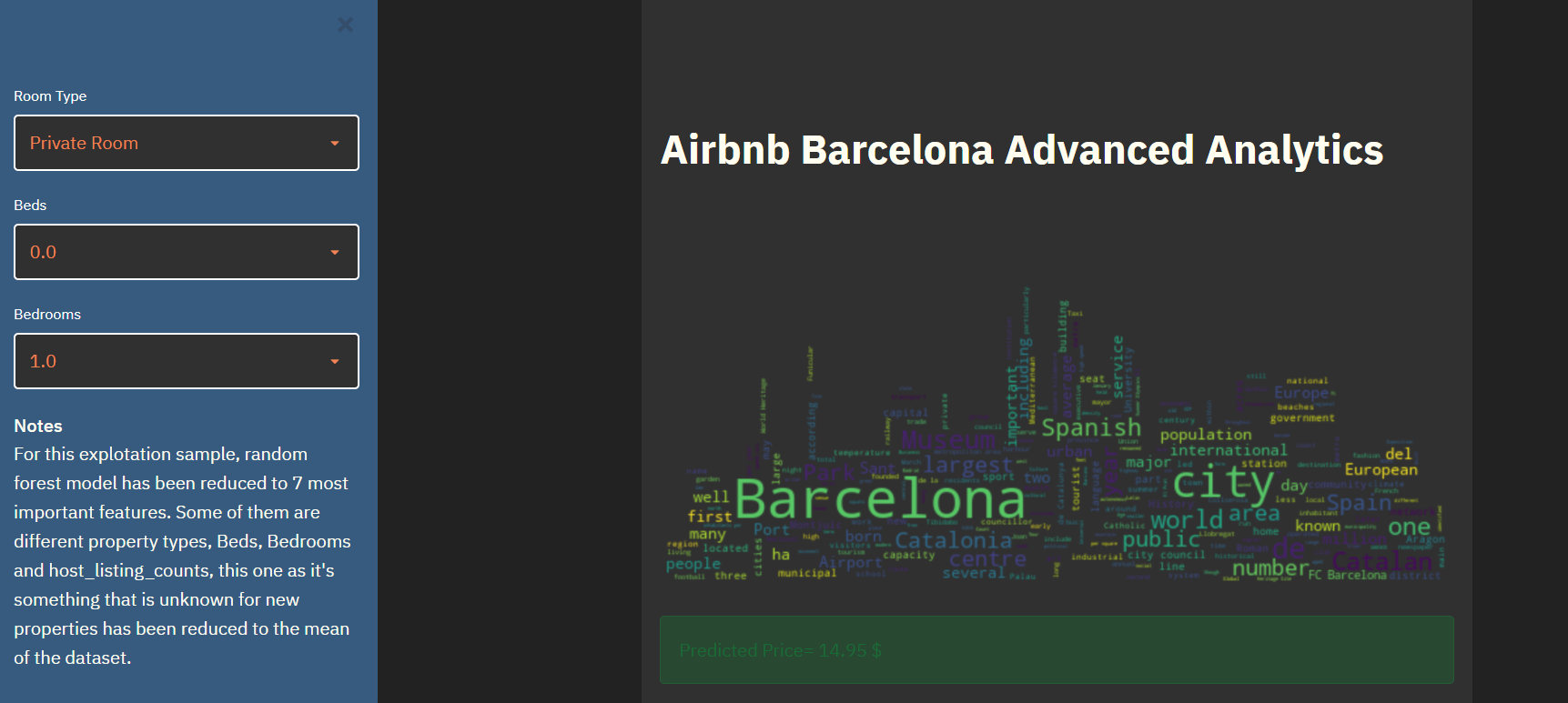# Barcelona Airbnb DS analysis (1st part)

## Source of data

Inside Airbnb

Date: 12/09/2020

\
|--Airbnb_Barcelona.ipynb
|--data
|-calendarBarcelona.csv
|-listingsBarcelona.csv

#Datamining library
import pandas as pd
import seaborn as sns
import numpy as np

from plotnine import *

#Reading Raw Data
airbnb_pub = raw_data.copy()


## General Exploration and Data Adjustment

print("Shape: ",airbnb_pub.shape)
print("Column Information:",airbnb_pub.info())

Shape:  (20337, 74)
<class 'pandas.core.frame.DataFrame'>
RangeIndex: 20337 entries, 0 to 20336
Data columns (total 74 columns):
#   Column                                        Non-Null Count  Dtype
---  ------                                        --------------  -----
0   id                                            20337 non-null  int64
1   listing_url                                   20337 non-null  object
2   scrape_id                                     20337 non-null  int64
3   last_scraped                                  20337 non-null  object
4   name                                          20324 non-null  object
5   description                                   20185 non-null  object
6   neighborhood_overview                         12447 non-null  object
7   picture_url                                   20337 non-null  object
8   host_id                                       20337 non-null  int64
9   host_url                                      20337 non-null  object
10  host_name                                     20330 non-null  object
11  host_since                                    20330 non-null  object
12  host_location                                 20305 non-null  object
14  host_response_time                            13233 non-null  object
15  host_response_rate                            13233 non-null  object
16  host_acceptance_rate                          16752 non-null  object
17  host_is_superhost                             20330 non-null  object
18  host_thumbnail_url                            20330 non-null  object
19  host_picture_url                              20330 non-null  object
20  host_neighbourhood                            14891 non-null  object
21  host_listings_count                           20330 non-null  float64
22  host_total_listings_count                     20330 non-null  float64
23  host_verifications                            20337 non-null  object
24  host_has_profile_pic                          20330 non-null  object
25  host_identity_verified                        20330 non-null  object
26  neighbourhood                                 12447 non-null  object
27  neighbourhood_cleansed                        20337 non-null  object
28  neighbourhood_group_cleansed                  20337 non-null  object
29  latitude                                      20337 non-null  float64
30  longitude                                     20337 non-null  float64
31  property_type                                 20337 non-null  object
32  room_type                                     20337 non-null  object
33  accommodates                                  20337 non-null  int64
34  bathrooms                                     0 non-null      float64
35  bathrooms_text                                20326 non-null  object
36  bedrooms                                      19630 non-null  float64
37  beds                                          19923 non-null  float64
38  amenities                                     20337 non-null  object
39  price                                         20337 non-null  object
40  minimum_nights                                20337 non-null  int64
41  maximum_nights                                20337 non-null  int64
42  minimum_minimum_nights                        20337 non-null  int64
43  maximum_minimum_nights                        20337 non-null  int64
44  minimum_maximum_nights                        20337 non-null  int64
45  maximum_maximum_nights                        20337 non-null  int64
46  minimum_nights_avg_ntm                        20337 non-null  float64
47  maximum_nights_avg_ntm                        20337 non-null  float64
48  calendar_updated                              0 non-null      float64
49  has_availability                              20337 non-null  object
50  availability_30                               20337 non-null  int64
51  availability_60                               20337 non-null  int64
52  availability_90                               20337 non-null  int64
53  availability_365                              20337 non-null  int64
54  calendar_last_scraped                         20337 non-null  object
55  number_of_reviews                             20337 non-null  int64
56  number_of_reviews_ltm                         20337 non-null  int64
57  number_of_reviews_l30d                        20337 non-null  int64
58  first_review                                  14526 non-null  object
59  last_review                                   14526 non-null  object
60  review_scores_rating                          14278 non-null  float64
61  review_scores_accuracy                        14267 non-null  float64
62  review_scores_cleanliness                     14269 non-null  float64
63  review_scores_checkin                         14263 non-null  float64
64  review_scores_communication                   14270 non-null  float64
65  review_scores_location                        14264 non-null  float64
66  review_scores_value                           14263 non-null  float64
68  instant_bookable                              20337 non-null  object
69  calculated_host_listings_count                20337 non-null  int64
70  calculated_host_listings_count_entire_homes   20337 non-null  int64
71  calculated_host_listings_count_private_rooms  20337 non-null  int64
72  calculated_host_listings_count_shared_rooms   20337 non-null  int64
73  reviews_per_month                             14526 non-null  float64
dtypes: float64(18), int64(21), object(35)
memory usage: 11.5+ MB
Column Information: None


here it's going to be detected/reviewed/changed:

• Aggregated columns, could be images, hashtags, datasets inside principal dataset, etc..
• Types that for our interest should be changed.
• False/True Values to Boolean ones
• String types that are numeric
• Manage Nan Values
• Resize using analytical and functional logic

### Currency detected as string (object) in spite of numbers (float)

If we look at our column information we will see that price is an 'object' type:

39  price                                         20337 non-null  object


Let's take a look to the column

airbnb_pub['price'].head(2)

0     $80.00 1$200.00
Name: price, dtype: object


It has been selected to string because it has $symbol. So we will need to modify by clearing this symbol and changing to float. airbnb_pub['price'] = airbnb_pub['price'].str.replace("$","").str.replace(",","").astype(float)
airbnb_pub['price'].dtype

dtype('float64')

airbnb_pub.price.describe()

count    20337.00000
mean        84.79339
std        210.66629
min          0.00000
25%         35.00000
50%         55.00000
75%         93.00000
max      10000.00000
Name: price, dtype: float64


Looking at percentiles we could see that more or less all values are in a range near 100, and then it seems that we have some outliers, we will try to 'catch' the normal behavior so we want to use and truncate data with most usual values.

If we 'graph' them it will help us to understand distribution.

(
ggplot(airbnb_pub,aes(x='id',y='price')) +
geom_boxplot(fill=orange,color='red') +
orange_dark_theme +
labs(x='',y='Price $') + theme(axis_text_x=element_blank())+ labs(title='Fig 1 - Raw price distribution of the dataset') )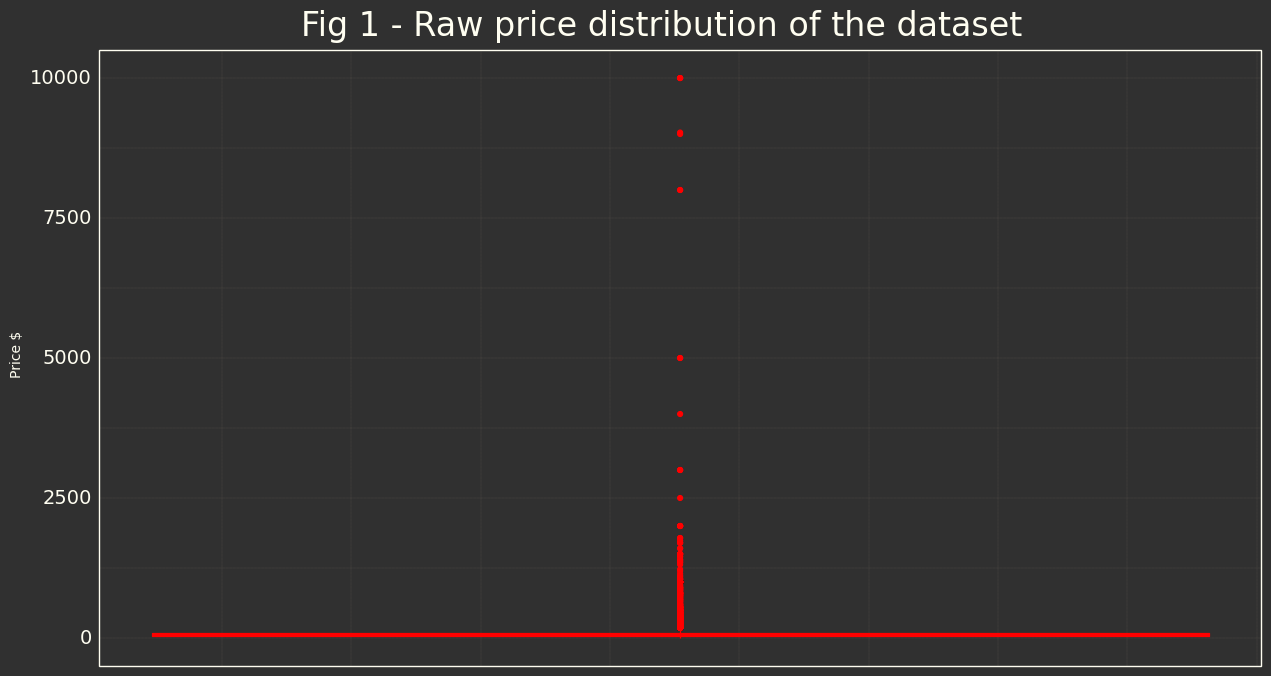Now the price filter is going to be changed till we have some values that belongs to the 'normal' expected price, to try to avoid in our future model any inconsistence because outliers. Here you have the last result, I have let some outliers to let the future model to detect some trend or non obvious behavior. airbnb_pub = airbnb_pub.loc[(airbnb_pub.price <=150) & (airbnb_pub.price>0)] ( ggplot(airbnb_pub,aes(x='id',y='price')) + geom_boxplot(fill=orange,color='red') + orange_dark_theme + labs(x='',y='Price$') +
theme(axis_text_x=element_blank()) +
labs(title='Fig 2 - Filtered price distribution of the dataset')
).draw();
print(airbnb_pub.price.describe())

count    18466.000000
mean        58.502799
std         33.610651
min          8.000000
25%         32.000000
50%         50.000000
75%         80.000000
max        150.000000
Name: price, dtype: float64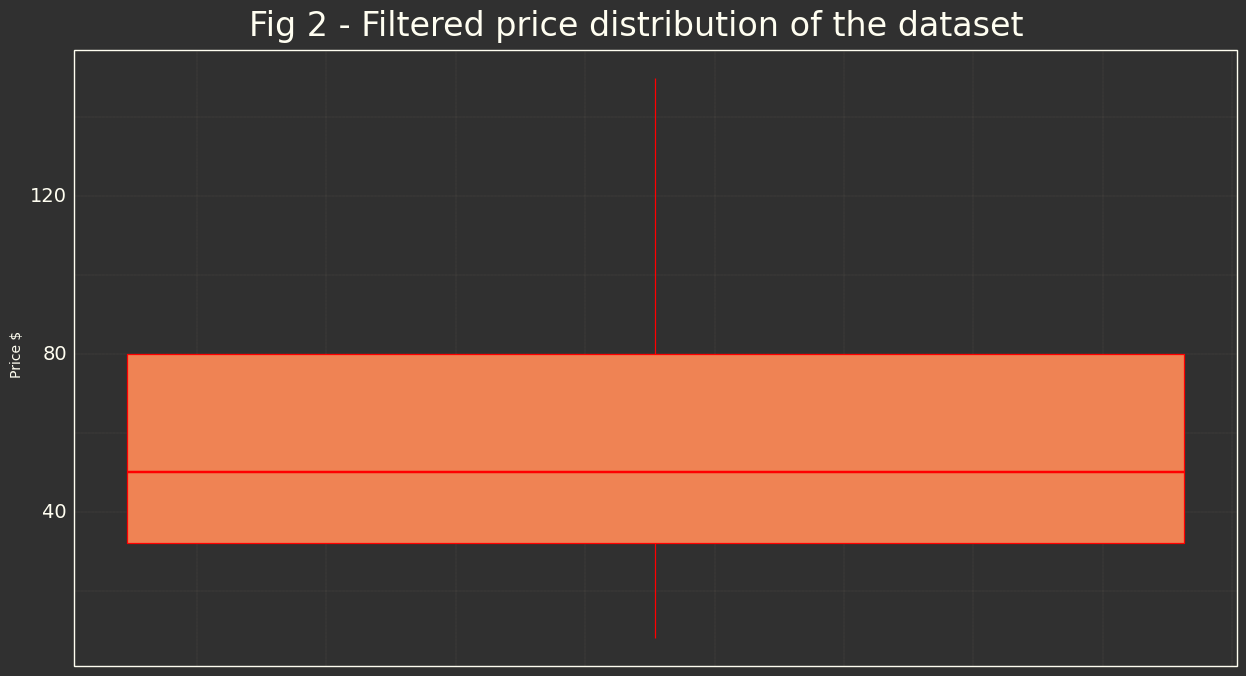Let's check only for understanding if we have normal distribution for this field.

(
ggplot(airbnb_pub,aes(sample='price')) +
geom_qq(color=orange) +
stat_qq_line(color=light_orange) +
labs(title='Fig 3 - QQPlot for Price Distribution')
)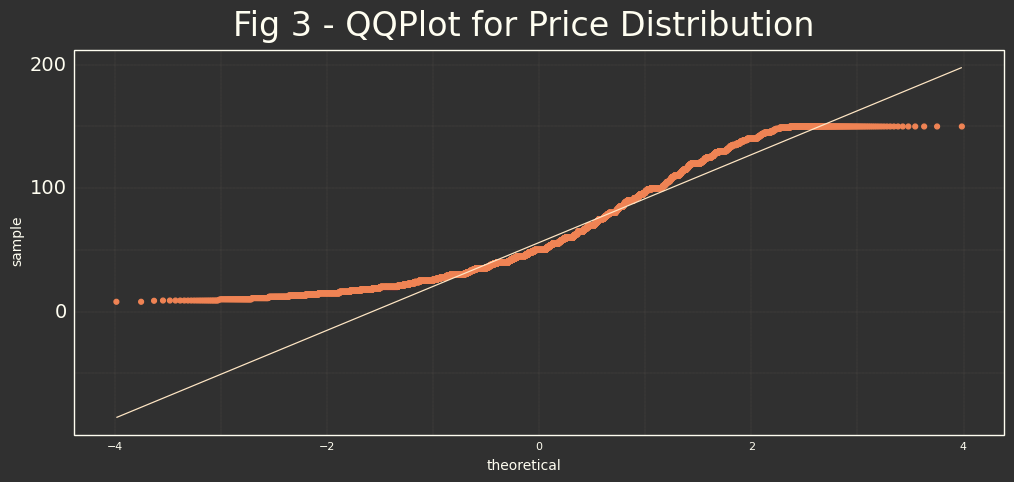As we know because we have done ^^U, we have normal distribution manually limited, and scored to the left. This will be important if in next parts we need to use some model impacted by normality of predicted value.

After that we have now smaller data collection without outliers, so we could think that we are going to take the usual behavior of the dataset in 95% of confidence.

### Extracting additional data from 'complex' fields

There are some data that is composed by different other data, we want to use them as it seems important for price determination, so we need to transform this information in new columns, we will start by creating data frame with all these data.

airbnb_pub.amenities.head(3)

0    ["Carbon monoxide alarm", "Fire extinguisher",...
3    ["Stove", "Microwave", "Refrigerator", "Oven",...
4    ["Refrigerator", "Microwave", "Carbon monoxide...
Name: amenities, dtype: object

#Text to column tool -> Vectorizer
from sklearn.feature_extraction.text import CountVectorizer
count_vectorizer = CountVectorizer(tokenizer=lambda x: x.split(','))
amenities = count_vectorizer.fit_transform(airbnb_pub.amenities)

#We are going to clean column names
columns=count_vectorizer.get_feature_names()
for index, item in enumerate(columns):
replaced = item.replace('"','').replace('[','').replace(']','').strip()
columns[index] = replaced

df_amenities = pd.DataFrame(amenities.toarray(),columns=columns)
df_amenities.drop('',axis=1,inplace=True)


After this process there are some duplicated columns that I will deal by checking if someone has true value, if yes, then it will select for the final value, and drop duplicates.

df_repeated = pd.DataFrame()
print('Starting amenities files, columns: ',df_amenities.shape)
for col in df_amenities.columns:
if col not in df_repeated.columns:
if len(df_amenities.filter(like=col).columns) > 1:
df_repeated[col] = df_amenities[col].any(1)
df_repeated = df_repeated.applymap(lambda x: 1 if x else 0)
df_amenities.drop(col,axis=1,inplace=True)
df_amenities = pd.concat([df_amenities,df_repeated],axis=1,join='inner')
df_amenities.columns = df_amenities.columns.str.replace(' ', '_')
print('Final amenities files, columns: ',df_amenities.shape)

Starting amenities files, columns:  (18466, 205)
Final amenities files, columns:  (18466, 106)


We have remove $>90$ repeated columns which will help to our model to be more efficient. Amenities looks like:

df_amenities.head(2)

baby_bath baby_monitor ... smart_lock smoke_alarm waterfront wifi window guards
0 0 0 ... 0 1 0 1 0
1 0 0 ... 0 0 0 1 0

2 rows x 106 columns

We will use at the end and add them to the dataset.

### Boolean literals to binary literals

In the visual exploration (most different possibilities excel/csviewer/spyder/vscode....) we have seen that some columns have 'T' for True and 'F' for false, we need to transform them in a way that we could use in future model.

# I'm going to 'catch' all possible columns to be transformed.
boolean_list = []
for col in airbnb_pub.columns:
if airbnb_pub[col].unique().size == 3:
column_array_values = np.delete(airbnb_pub[col].unique(),2,0)
if set(column_array_values)==set(['t','f']):
boolean_list.append(col)
if airbnb_pub[col].unique().size == 2:
if set(column_array_values)==set(['t','f']):
boolean_list.append(col)
boolean_list

['host_is_superhost',
'host_has_profile_pic',
'host_identity_verified',
'has_availability',
'instant_bookable']

# Transform to binary
for col in boolean_list:
airbnb_pub[col] = airbnb_pub[col].replace('f',0)
airbnb_pub[col] = airbnb_pub[col].replace('t',1)


### Neighbourhood could be used?

airbnb_pub.filter(like='neigh').info()

<class 'pandas.core.frame.DataFrame'>
Int64Index: 18466 entries, 0 to 20336
Data columns (total 5 columns):
#   Column                        Non-Null Count  Dtype
---  ------                        --------------  -----
0   neighborhood_overview         11264 non-null  object
1   host_neighbourhood            13472 non-null  object
2   neighbourhood                 11264 non-null  object
3   neighbourhood_cleansed        18466 non-null  object
4   neighbourhood_group_cleansed  18466 non-null  object
dtypes: object(5)
memory usage: 865.6+ KB


We will get neighbourhood_cleansed because it has more accurately location, it's subgroup of neighborhood_group_cleansed, and we could return, or re-categorize through this column any time we want. Both don't have nan values, so are perfect for our analysis.

### Looking for nan values in number columns

We have seen that we have different types in our dataset, in this case we want to look for numerical ones, we have only 2 types in our dataset that are numerical float64 and int64. We want to know if they are completed or have Nan values, if Nan then we want to change with the mean of the Serie (column).

Notice that false true changed to boolean could be also affected

for c in airbnb_pub:
if ((airbnb_pub[c].dtype=='float64') | (airbnb_pub[c].dtype=='int64')):
if airbnb_pub[c].isnull().any():
airbnb_pub[c] = airbnb_pub[c].fillna(airbnb_pub[c].median())
for col in airbnb_pub.columns:
if airbnb_pub[col].size == airbnb_pub[col].isnull().sum():
airbnb_pub.drop(col,axis=1,inplace=True)


### Calculated field

Location could be something important in price calculation (near to the beach, good neighbourhood, etc..), to have quick metric valuation 2D location is going to be transformed to 1D location variable by adding the 2 values (could be any other operation).

airbnb_pub['location1d'] = airbnb_pub['latitude'] + airbnb_pub['longitude']


Summary

• We have detected some pricing data that initially was selected as string and we have changed it to numeric format.
• Resize our dataset by clearing all negative prices and outliers prices.
• All string Boolean values have been changed to numeric ones.
• All Nan numeric dataset values have been treated to avoid any numerical issue
• Some data items are dataset itself, we have caught it, split and transform in an individual dataset
• Checked if some feature could be interesting and validate it (nan, etc..)

We have to take some decision about which data will be relevant for our model, which columns, thinking that our objective is to have a model that predicts or help us which values are most relevant in price determination.

We will also add our amenities dataset as we found them relevant for price determination, and we want to have them in our model.

#Small script to let me select in the next step the columns easylly using notebook :P
#I have cleared the output to readability reasons.
for c in airbnb_pub.columns:
print('"',c,'",',sep="")

"id",
"listing_url",
"scrape_id",
"...",
"calculated_host_listings_count_private_rooms",
"calculated_host_listings_count_shared_rooms",
"reviews_per_month",
"location1d",

airbnb_pub = airbnb_pub[[
"id",
"host_is_superhost",
"host_identity_verified",
"host_has_profile_pic",
"instant_bookable",
"bedrooms",
"room_type",
"beds",
"neighbourhood_cleansed",
"property_type",
"number_of_reviews",
"host_listings_count",
"host_total_listings_count",
"minimum_nights",
"review_scores_rating",
"latitude",
"longitude",
"location1d",
"price"
]]

airbnb_data = pd.concat([airbnb_pub,df_amenities],axis=1,join='inner')
airbnb_data.to_csv("./data/output.csv")
print("Final output dataset Shape: ",airbnb_data.shape)

Final output dataset Shape:  (16732, 125)


And that's all, airbnb data cleaning has been done and we are ready for analyze the data that we 'feel' that is relevant for pricing, of course, using the same pipeline we could test/check and re-catch all the steps that we have done to review or try or remove some data with the results we have when modeling or analyzing the data.

# Barcelona Airbnb DS analysis (2nd part)

We are going now to try to understand our data a bit more.

## Importing Libraries

#Data
import pandas as pd

#Graphics libraries
from plotnine import *
import seaborn as sns
import matplotlib.pyplot as plt


At the end of part1 we finished with cleaned data set output.csv, we will continue using this data set, se we will read it, adjust a little bit dropping some garbage columns, and go on.

airbnb_data = pd.read_csv("./data/output.csv")
airbnb_data.drop(airbnb_data.filter(like='Unnamed').columns,axis=1,inplace=True)


## Data Visualization

We will start trying to find correlations/aggrupations in a visual way, for that we have an excelent tool from seaborn:

sns.pairplot(airbnb_data[[
"host_is_superhost",
"host_identity_verified",
"host_has_profile_pic",
"instant_bookable",
"bedrooms",
"room_type",
"beds",
"neighbourhood_cleansed",
"property_type",
"number_of_reviews",
"host_listings_count",
"minimum_nights",
"review_scores_rating",
"price"
]],hue='property_type')
# Title
plt.suptitle("Fig 1 - Correlation between principal variables",  y=1.02, size = 28);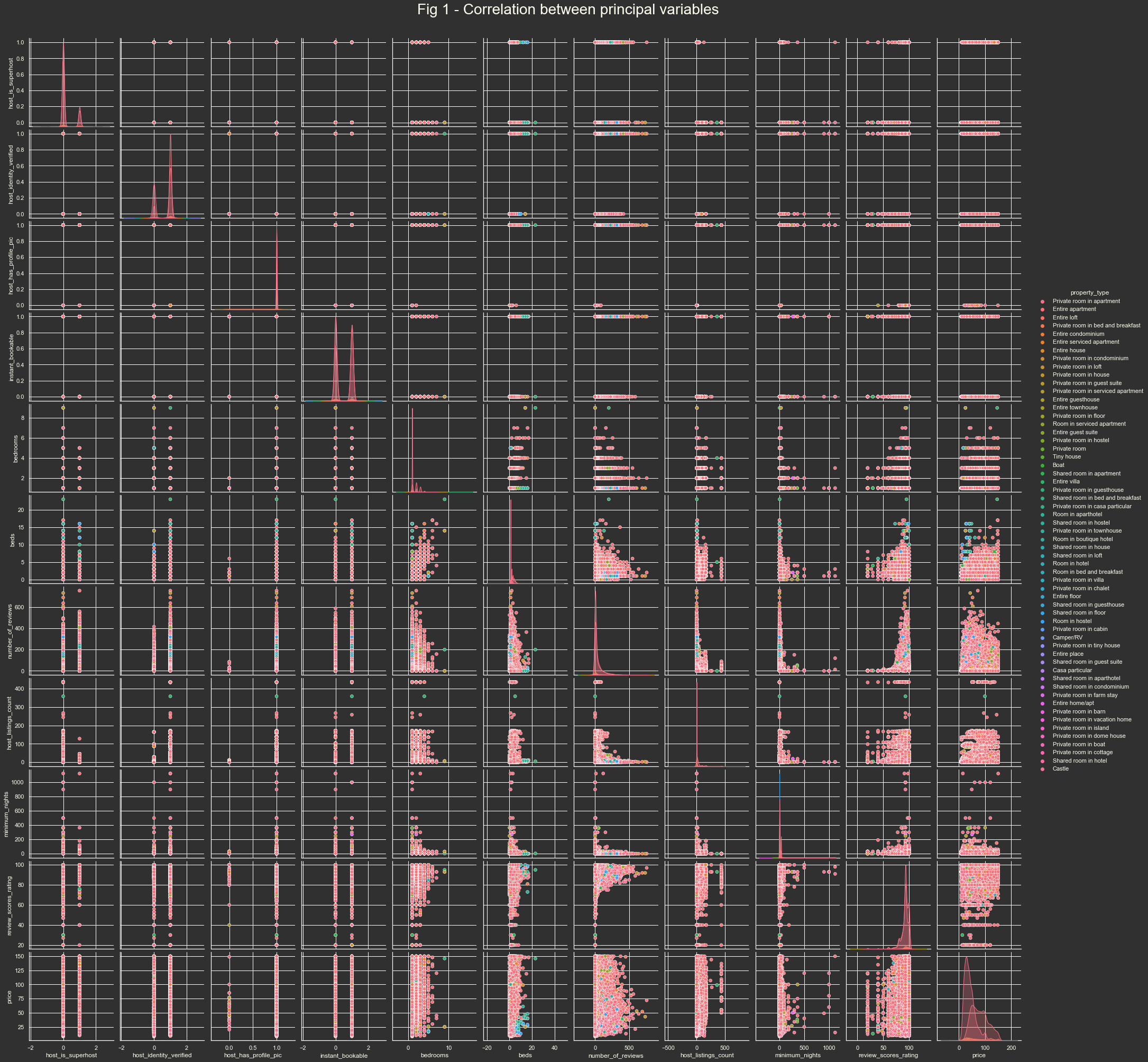In this type of graphs, we are trying to find some logic that helps us understand how our data is distributed, if there is some correlation, and also if it's obvoius some aggrupation. To follow the discussion, I encourage to see this graphic in a separate window and maximized. Pairplot Image

We have 2 types of graphics:

• Crossed characteristics: plot graphic with the relation with the 2 variables.
• Diagonal characteristics: As we have the same 2 characteristics, here we found distribution plot of our characteristics.

All of them are colored by property type, as it's clear that is an important aggregation characteristic which I will try to highlight in any graphic so we could analyze all data with this dimension disaggregated.

Some conclusions:

• There are some features that have different distribution related to the price, it's not clear the relation and how important it is, but for example number of reviews vs price, you could see that it's not rectangle (normal distribution) it has some values when you have lots of reviews that seems to 'change' the price or some relation exists.
• The properties which have more reviews usually have also best scores.
• Score rating doesn't seem clear that impacts over the price, as we have lots of different prices distributed the same way in all the score range.
• We have several 1 bedroom with 1 bed publication.
• It seems in general that people review and rate similar way...

### Review Scores Rating

Let's see one particular distribution, scores rating, you will see in Fig 2 that we have more or less always 80-100 rating with really few scores under that. It could mean that people that doesn't like some property it's not giving any comment, and who has enjoyed the property usually scores it.

It's not clear how could affect to our predicted price and if there are some other categories most relevant. The relation with score and price exists, because you found more expensive properties when looking for best scores, but there are also inexpensive ones. Fig 3

(
ggplot(airbnb_data, aes(x='review_scores_rating')) +
geom_density(aes(y='stat(count)'), fill=orange,color='red',alpha=0.3) +
geom_bar(aes(y='stat(count)'),fill=yellow_orange,alpha=0.2) +
orange_dark_theme +
labs(x='Score 0 - 100',y='Number of Publications',title='Fig 2 - Score Review Distribution')
)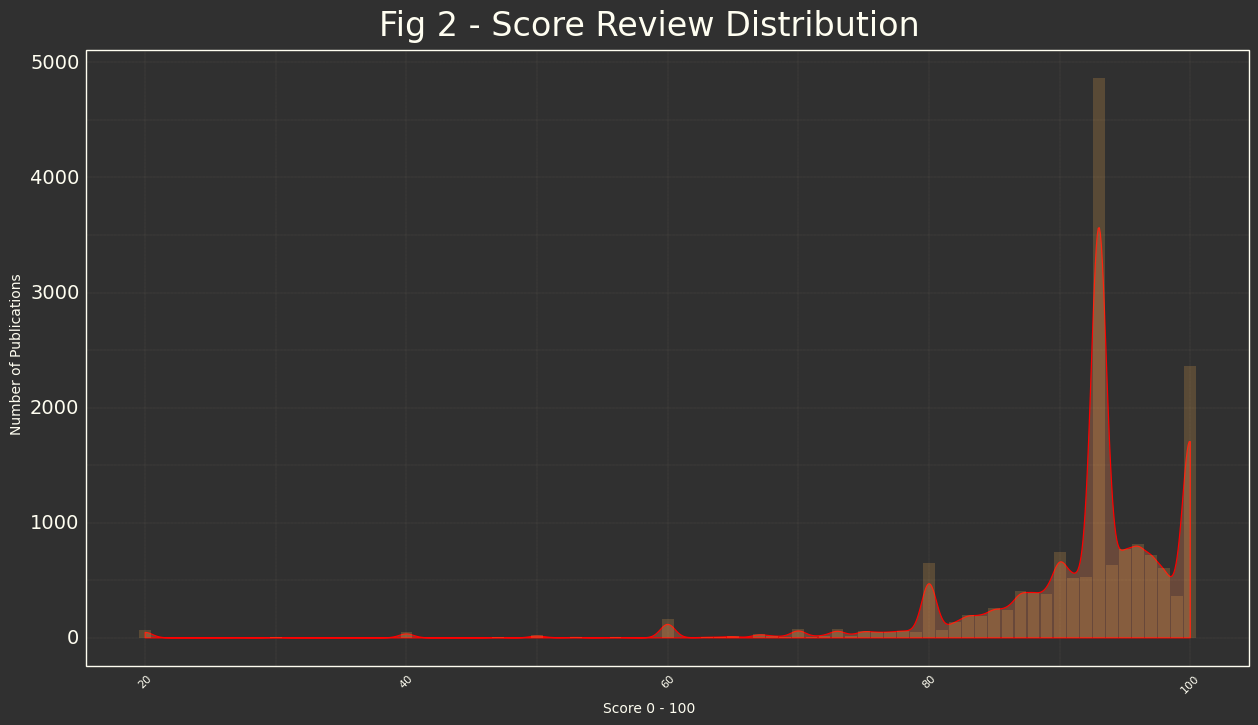(
ggplot(airbnb_data[airbnb_data['property_type']=='Private room in apartment'],
aes(x='review_scores_rating',y='price')) +
geom_point(aes(color='price')) +
scale_color_distiller(palette= 'Oranges') +
orange_dark_theme +
labs(x='Score 0 - 100',y='Price $',title='Fig 3 - Price/Score Distribution') )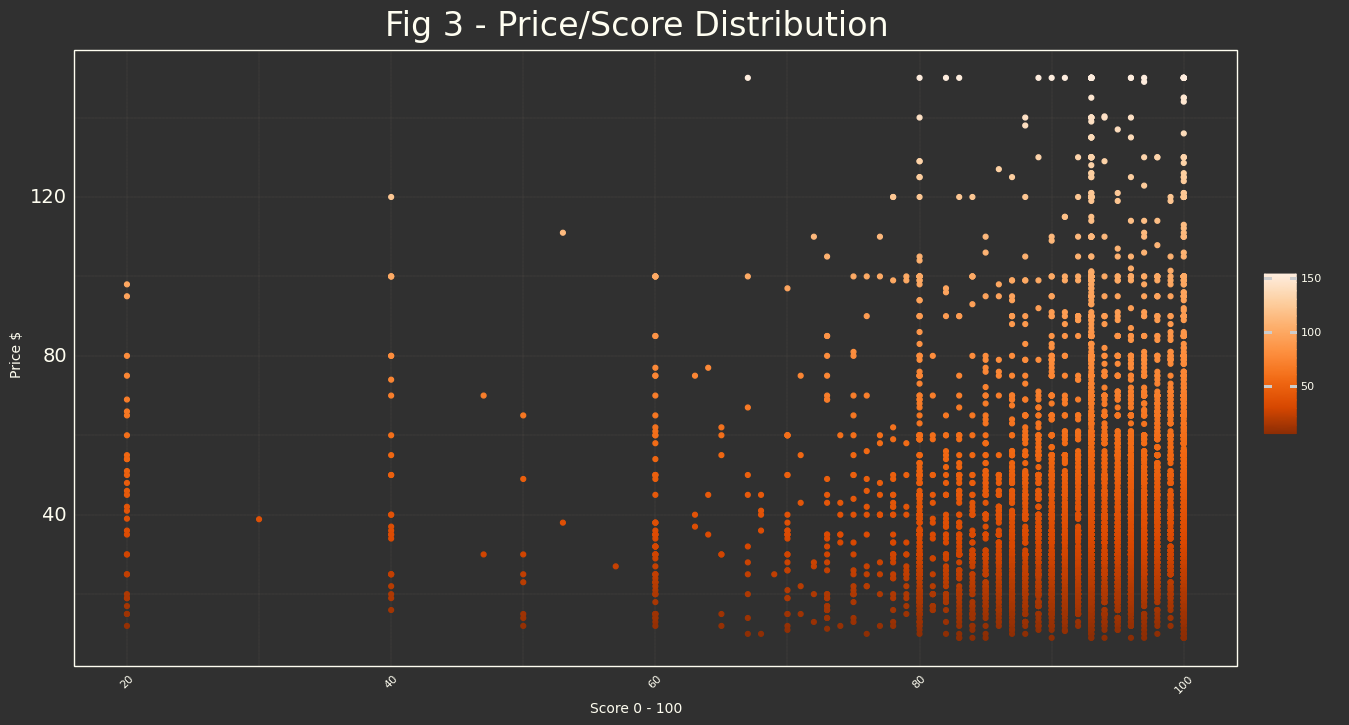### Property Type We are working all the time disaggregating by property_type, this is because from an intuitive point of view, it's clear that room it's not the same as house, so this variable will be relevant in price determination. We can see which is the price distribution for each property type, to confirm our intuitive interpretation. sorted_by_price = airbnb_data.groupby(by='property_type')['price'].median().sort_values(ascending=False).index  ( ggplot(airbnb_data,aes(x='property_type',y='price',fill='property_type',group='property_type')) + scale_x_discrete(limits=sorted_by_price) + geom_boxplot(color=light_orange,show_legend=False) + labs(x='',y='Price$',title='Fig 4 - Price distribution aggregated by Property Type') +
orange_dark_theme +
theme(
axis_text_x=element_text(angle=90, color =light_white, size=8),
)
)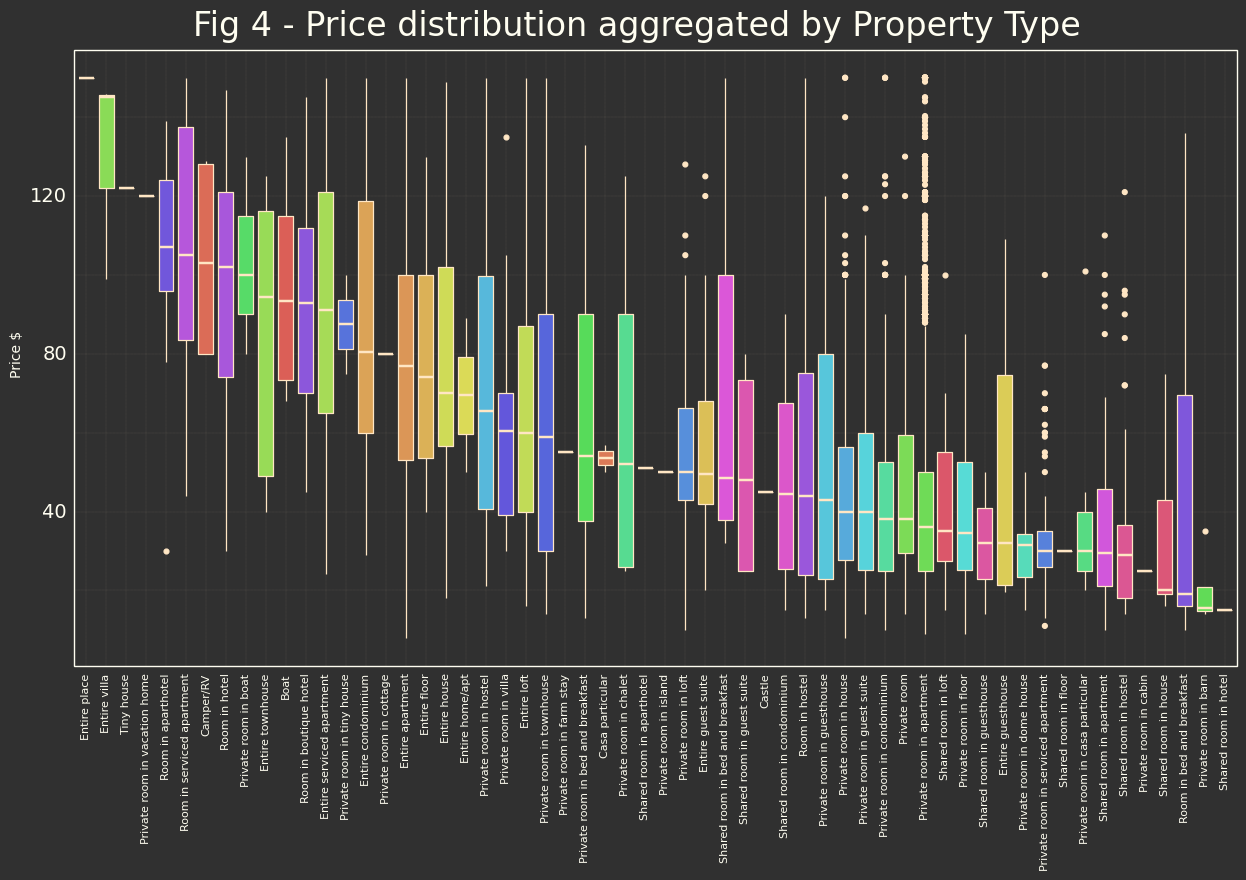And we could check also which is the top 10 types of our dataset.

sorted_by_price_top10 = airbnb_data.groupby(by='property_type')['price'].count().sort_values(ascending=False).head(10).index
(
ggplot(airbnb_data,aes(x='property_type',y='id',fill='property_type',group='property_type')) +
scale_x_discrete(limits=sorted_by_price_top10) +
scale_fill_brewer(palette = "Oranges") +
geom_bar(aes(y='stat(count)'),color=light_orange,show_legend=False) +
labs(x='',y='Number of Publications',title='Fig 5 - Top 10 Property Type Publications') +
orange_dark_theme +
theme(
axis_text_x=element_text(angle=45, ha='right',color =light_white, size=12),
)
)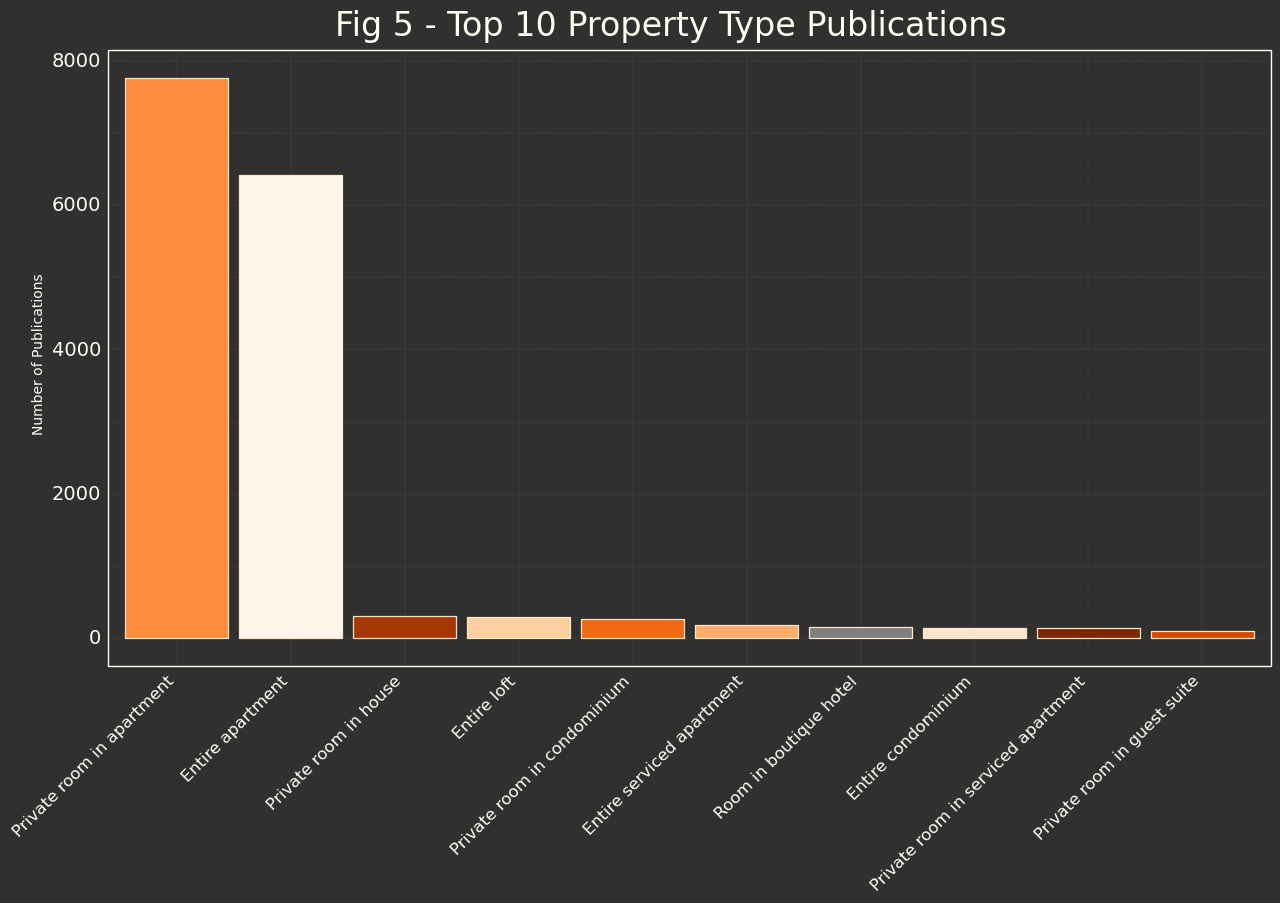### Neighbourhood

And what about the neighbourhoods? Let's take a look.

sorted_by_price = airbnb_data.groupby(by='neighbourhood_cleansed')['price'].median().sort_values(ascending=False).index

(
ggplot(airbnb_data,aes(x='neighbourhood_cleansed',y='price',fill='neighbourhood_cleansed',group='neighbourhood_cleansed')) +
scale_x_discrete(limits=sorted_by_price) +
geom_boxplot(color=light_orange,show_legend=False) +
labs(x='',y='Price $',title='Fig 6 - Price distribution aggregated by Neighbourhood') + orange_dark_theme + theme( axis_text_x=element_text(angle=90, color =light_white, size=8), ) )sorted_by_price_top10 = airbnb_data.groupby(by='neighbourhood_cleansed')['price'].count().sort_values(ascending=False).head(10).index ( ggplot(airbnb_data,aes(x='neighbourhood_cleansed',y='id',fill='neighbourhood_cleansed',group='neighbourhood_cleansed')) + scale_x_discrete(limits=sorted_by_price_top10) + scale_fill_brewer(palette = "Oranges") + geom_bar(aes(y='stat(count)'),color=light_orange,show_legend=False) + labs(x='',y='Number of Publications',title='Fig 7 - Top 10 Neighbourhood Publications') + orange_dark_theme + theme( axis_text_x=element_text(angle=45, ha='right',color =light_white, size=12), ) )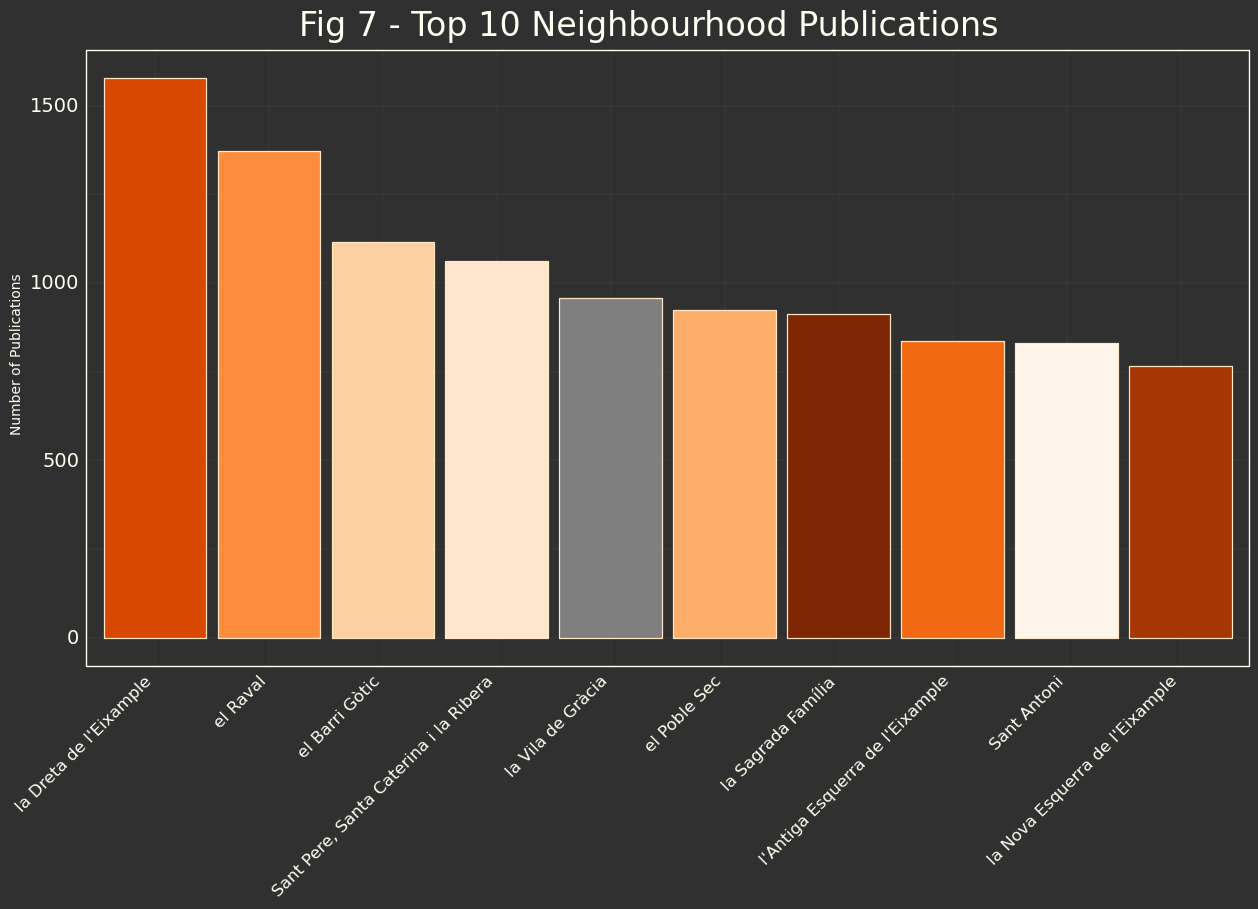It seems logic, because these neighbourhoods are the most typical and touristics. We have seen also that there is some type of relation (with lots of distributions and casuistics) but in fact mean price relation between touristics/business neighbourhoods and more usual/blue neck neighbourhoods. ### Correlations Now we are going to check all the correlations from our dataset, so we could avoid using the correlated ones, as they could be described one from the other and when modeling we need only independent variables. df_corr = airbnb_data.corr() df_corr = df_corr[(df_corr>0.8) | (df_corr<-0.8)] #I want only most relevant correlations. # For visualization I don't want all nan values expect diagonal = 1 # So I will clear all not relevant ones. df_corr = df_corr.abs() df_corr['Corr'] = df_corr.sum() df_corr = df_corr[df_corr['Corr'] > 1] df_corr.drop('Corr',axis=1,inplace=True) #calculation column df_corr.dropna(1,how='all',inplace=True) #All full nan columns  sns.heatmap(df_corr,cmap='magma'); plt.suptitle("Fig 8 - Correlation Dependencies between principal variables", size = 24);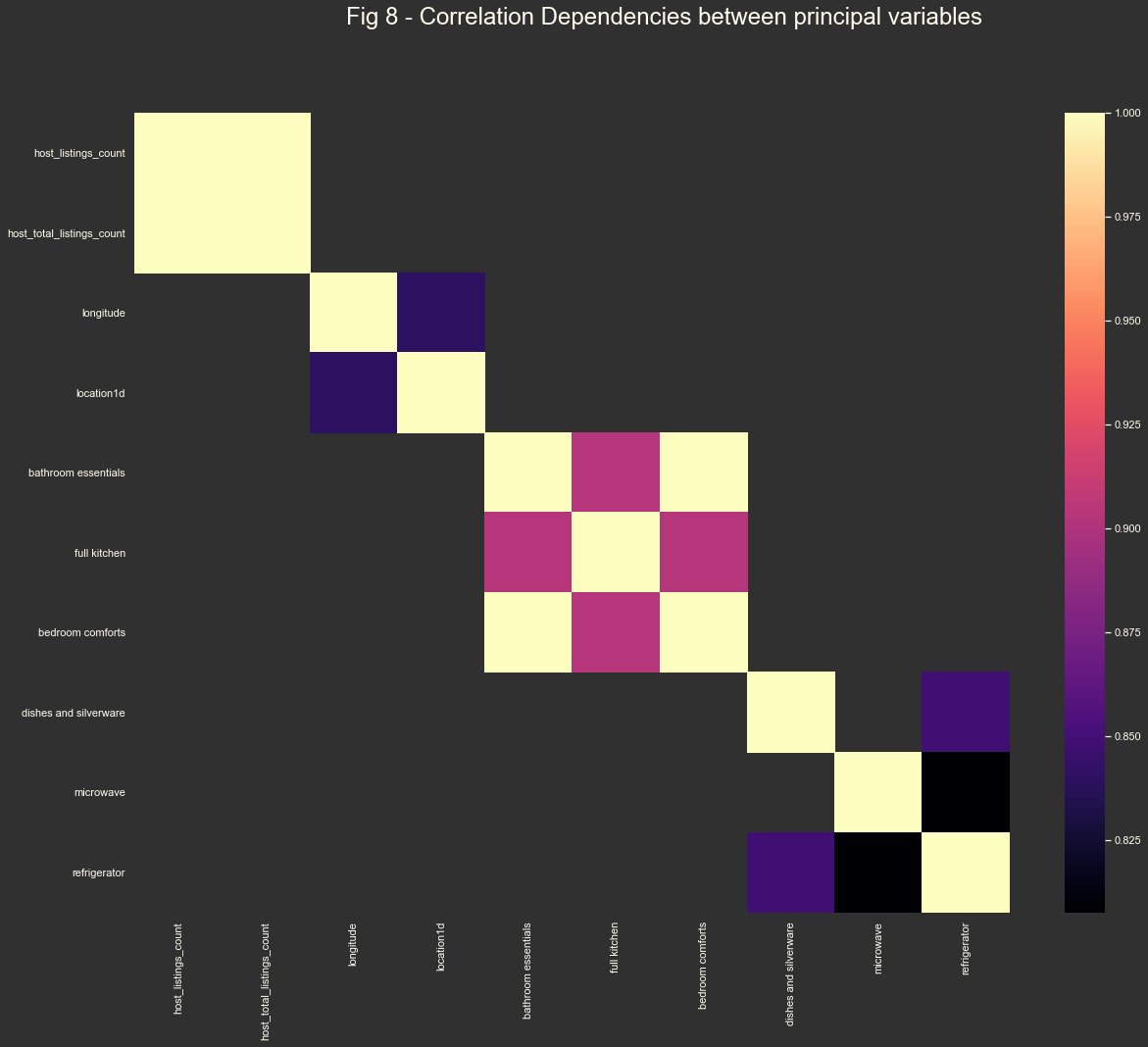We have cleaned >0.8 values, but we could drop till 0.95, but if some of them are 'logic' we could drop also <0.95 value Conclusions: • We have that if we have soap we have bath essentials so we could drop one of them. • 'host_listings_count' and 'host_total_listings_count' are highly related. • 'bedrooms essentials' and 'bedrooms comforts' and kitchen are related. • 'refrigerator' and 'microwave' are related. • If we have some comfort characteristic it seems that is shared with other comfort characteristics (bedroom, with kitchen, but we will let them. • Of course, location1d and latitude are related as one has been done with the other. All related features will be removed and let only 1 when modeling. ### Location analysis Remember, we have created variable called 'location1d' which is the sumatory of latitude and longitude that will help us to determinate 1 point We need to see which is the behavior for location, so we will wrap our graphics with the property type, and its relation with location. Let's take a look: ( ggplot(airbnb_data, aes(x='price',y='location1d')) + geom_point(aes(color='price')) + #it's not necessary color by price but help visualization facet_wrap('property_type', ncol = 3) + orange_dark_theme + theme( figure_size=(20,70) ) + labs(x='Price$',
y='Location (Lat+Long)',
title='Fig 9 - Location vs Price aggregated by Property Type')
)Looking at 'Private room in apartment' and 'entire apartment' we found some correlation between locations and prices, we could see triangle (clear in 'Private room in apartment') where some locations have expensive distribution (you have lots of points to the right) compared with other locations. Same figure in 'entire apartment' where prices are more distributed but for the same locations you have several expensive points.

Is possible that other types follow the same behavior 'Private room in house' for example, but I think that we don't have enough data to arrive to some conclusion.

In fact, the impact over the price it's going to be very little because the big density of the other prices which are more or less distributed randomly so it makes us think that other variables will be much relevant for price determination.

#### (BONUS) We will focus on the most usual publication and take a more detailed view

airbnb_private = airbnb_data[['latitude','longitude','price']][airbnb_data.property_type == 'Private room in apartment']
airbnb_private.price = airbnb_private.price.astype(float)

#Centering the map
init_lat = airbnb_private.latitude.mean()
init_long = airbnb_private.longitude.mean()


We are going to represent in a map:

• Heatmap: Which represents 'heat' points for density publication.
• Clustering: Publications density.
• Color Markers: Represent different prices.
import folium
from folium.plugins import MarkerCluster, HeatMap
m = folium.Map(
tiles='CartoDB Dark_Matter',
location=[init_lat, init_long],
zoom_start=13,
max_bounds=True
)
for index, row in airbnb_data.iterrows():
lat_air = row.latitude
long_air = row.longitude
price = row.price
if (price > 0 ) & (price < 30):
folium.Marker([lat_air, long_air],
tooltip=price,
icon=folium.Icon(color='green'),
clustered_marker = True
elif (price < 50) & (price >= 30):
folium.Marker([lat_air, long_air],
clustered_marker = True,
tooltip=price,
icon=folium.Icon(color='orange')
elif (price < 90) & (price >= 50):
folium.Marker([lat_air, long_air],
clustered_marker = True,
tooltip=price,
icon=folium.Icon(color='lightred')
else:
folium.Marker([lat_air, long_air],
clustered_marker = True,
tooltip=price,
icon=folium.Icon(color='red')

# convert to (n, 2) nd-array format for heatmap
privateArr = airbnb_data[['latitude', 'longitude']].values

# plot heatmap


### Link to the Airbnb Map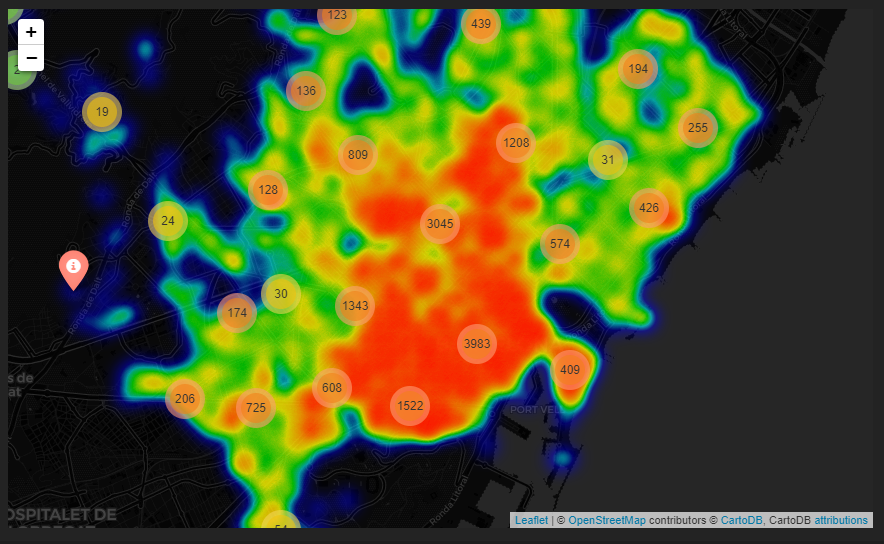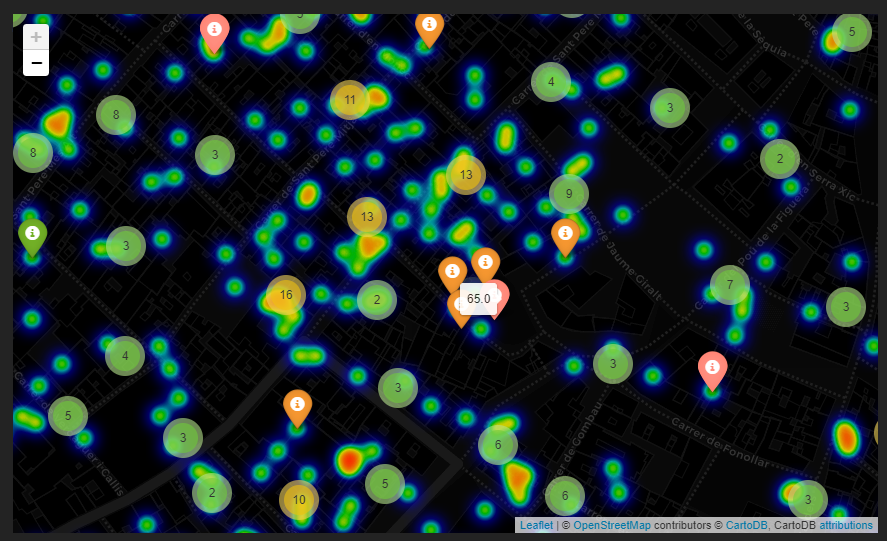# Barcelona Airbnb DS analysis (3rd part)

\
|--Airbnb_Barcelona.ipynb
|--data
|   |-output.csv
|--model
|-randforestKK_NNNN.joblib


Here we are going to model our data to perform the best result we could and then save the model to use in a production system as a reference and consumable service

## Importing Libraries

#Data
import pandas as pd
import numpy as np

#Graphics libraries
from plotnine import *
import seaborn as sns
import matplotlib.pyplot as plt

#Librearies for ML model
from sklearn.model_selection import train_test_split
from sklearn.ensemble import RandomForestRegressor
from sklearn.tree import DecisionTreeRegressor
from xgboost import XGBRegressor, to_graphviz
from sklearn.feature_selection import SelectKBest, f_regression
#Accuracy
from sklearn.metrics import r2_score, mean_squared_error, mean_absolute_error
from sklearn.metrics import balanced_accuracy_score, roc_auc_score, make_scorer # for scoring during cross validation
from sklearn.model_selection import GridSearchCV # cross validation


At the end of part1 we finished with cleaned data set output.csv, we will continue using this data set, which is the same that we have analyzed in part2.

Correlations found in part2 will be droped.

Categorical data that we use as is in part2, here we will transform in columns and boolean data type, without loosing the information, it will be ready for model.

airbnb_data = pd.read_csv("./data/output.csv")
airbnb_data.drop(airbnb_data.filter(like='Unnamed').columns,axis=1,inplace=True)
airbnb_data.drop([
'id', #This was usefull for graphics analysis for samples, but not for modeling
'latitude',
'longitude',
'bedroom comforts', #bedroom essentials related
'host_total_listings_count', #related to host_listings_count
'microwave', #related to refrigerator
],axis=1,inplace=True)

# One-hot encode using pandas get_dummies
airbnb_data = pd.get_dummies(airbnb_data)


## Independent variable vs dependent variables

We are going to separate our dependent variable (the one we want to predict) from the independent values.

Xraw = airbnb_data.drop(['price'],axis=1).astype('float')
X = Xraw.copy()
y = airbnb_data['price'].astype('float')


## Random Forest Model

We are going to do regression model based on Random Forest algorithm. We are going to use this model based on:

• We have several columns/characteristics to be evaluated and this model it's good one to lots of columns regression (independent variables).

### Filter Selection

As we have more or less 200 variables to be analyzed, we could try to fit all them or try to find the most relevant ones. To do that we could select the best features based on univariate statistical tests, removing all but the $k$ highest scoring features.

Also, we could check how this $k$ feature impacts in our model score, so we would try to find the best combination of relevant features, which could let us to explain the model (in random forest is always difficult) and also make our model more efficient as it will do less calculation to good result.

results = []
count = 0
minim_abs = 99999999999
nmax = 9

N = X.shape
print('|----|---------------------|')
print('| k  |       RMSE          |')
for i in range (1,N):
X = Xraw.copy()
X = SelectKBest(f_regression,k=i).fit_transform(X,y)
X_train, X_test, y_train, y_test = train_test_split(X, y, test_size=0.30, random_state=42)
random_forest_regression = RandomForestRegressor()
random_forest_regression.fit(X_train,y_train)
y_test_pred = random_forest_regression.predict(X_test)
results.append((mean_squared_error(y_test,y_test_pred))**(1/2))
print('|----|---------------------|')
print('|',i,' | ',(mean_squared_error(y_test,y_test_pred))**(1/2),'|')
#Exit at nmax items not decreasing
if len(results) != 0:
if (mean_squared_error(y_test,y_test_pred))**(1/2) > minim_abs:
count += 1
else:
minim_abs = (mean_squared_error(y_test,y_test_pred))**(1/2)
count = 0
else:
count = 0
if count > nmax:
print('|----|---------------------|')
print('| breaking after nmax items|')
break
print('|----|---------------------|')

|----|---------------------|
| k  |       RMSE          |
|----|---------------------|
| 1  |  28.93177920365006 |
|----|---------------------|
| 2  |  28.897571906376317 |
|----|---------------------|
| .. |        ..           |
|----|---------------------|
| 78  |  23.28982612403383 |
|----|---------------------|
| 79  |  23.189823897435403 |
|----|---------------------|
| 80  |  23.31864216244052 |
|----|---------------------|
| 81  |  23.298398543690762 |
|----|---------------------|
| 82  |  23.246322375416927 |
|----|---------------------|
| 83  |  23.23919914973194 |
|----|---------------------|
| 84  |  23.255104654137757 |
|----|---------------------|
| 85  |  23.311416436432804 |
|----|---------------------|
| 86  |  23.27902654988726 |
|----|---------------------|
| 87  |  23.368225650619195 |
|----|---------------------|
| 88  |  23.370200396371878 |
|----|---------------------|
| breaking after nmax items|
|----|---------------------|

k=results.index(min(results)) + 1
print('Minimum Element in the list is',k,' and is ',min(results))

Minimum Element in the list is 79  and is  23.189823897435403


We have check model score for $k$ possibilities, in fact it has been checked some more, but this is possible final result, as it seems that some limit value has been find.

N = k + 10
x_elbow = np.arange(1,len(results)+1,1)
y_elbow = results
elbow = pd.DataFrame({'x':x_elbow,'y':y_elbow})
(
ggplot(elbow,aes(x='x',y='y'))+
geom_line(color=orange) +
scale_x_continuous( limits=(1,N), breaks=range(1,N,1))+
geom_vline(xintercept=k,color=yellow_orange) +
labs(x='k Number',y='RMSE',title='Fig 2 - Error vs "k" variable (LR)') +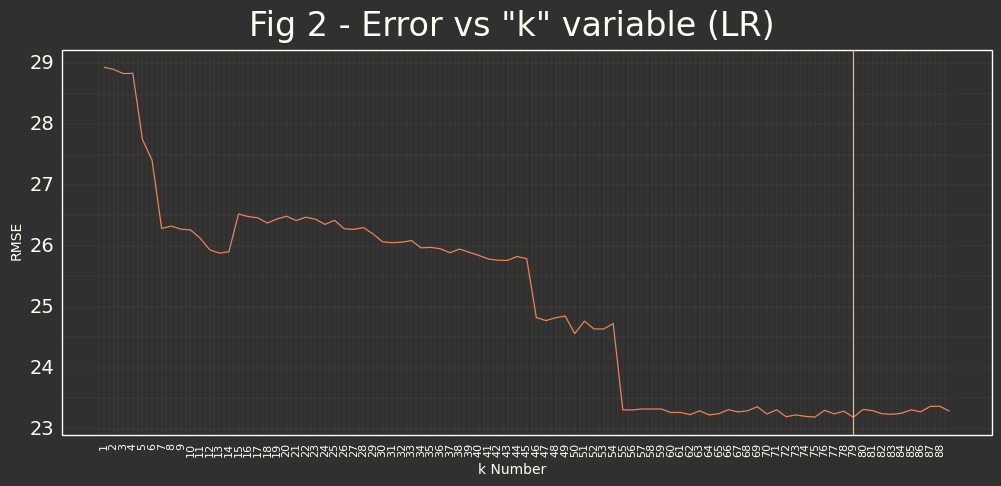We will take k= {{k}} as the minimum.

### Model pre-filtered and score

N_ESTIM = 1000
X = Xraw
selection = SelectKBest(f_regression,k=k)
X = selection.fit_transform(X,y)
X_train, X_test, y_train, y_test = train_test_split(X, y, test_size=0.30, random_state=42)
random_forest_regression = RandomForestRegressor(n_jobs=2,n_estimators=N_ESTIM, oob_score=True)
random_forest_regression.fit(X_train,y_train)
y_test_predict = random_forest_regression.predict(X_test)
y_train_predict = random_forest_regression.predict(X_train)
print('K filter value: ',k)
print('Number Random Forests: ',N_ESTIM)
rf_results=pd.DataFrame({'algorithm':['Random Forest'],
'Training error': [mean_absolute_error(y_train,y_train_predict)],
'Test error':[mean_absolute_error(y_test,y_test_predict)],
'Train_r2_score':[r2_score(y_train,y_train_predict)],
'Test_r2_Score':[r2_score(y_test,y_test_predict)]})
print(rf_results)

K filter value:  79
Number Random Forests:  1000
algorithm  Training error  Test error  Train_r2_score  Test_r2_Score
0  Random Forest        6.323665   17.327035        0.934937       0.529434

feat_imp=pd.DataFrame(random_forest_regression.feature_importances_,
index=Xraw.iloc[:,selection.get_support(indices=True)].columns,
columns=['Importance'])
feat_imp['feature'] = feat_imp.index
feat_imp_top10 = feat_imp.loc[feat_imp['Importance'].nlargest(10).index]
ax = sns.barplot(x="Importance", y="feature", data=feat_imp_top10,palette="Oranges")
# Title
plt.suptitle("Fig 3 - Random Forest Top10 Features",y=0.93, size = 24);Also we will try to have graphical view of how is distributed our predicted data from testing data.

result_df = pd.DataFrame({'Test Data':y_test,'Predicted Data':y_test_pred})
result_df.reset_index(drop=True,inplace=True)
result_df['id'] = result_df.index
result_df = pd.melt(result_df,id_vars='id', value_vars=['Test Data','Predicted Data'])

(
ggplot(result_df,aes(x='id',y='value',color='variable')) +
geom_point() +
scale_color_manual(values = ['#10D0EE',orange]) +
labs(title='Fig 4 - Predicted Data vs Test Data (RF)',x='',y='Price $',color='Data Type') + )Well$R^2=0,52$It's not a good result, but it's not the worst. Comparing predicted and testing data it seems that it has good behavior around the mean price of the dataset and it's not so good with the prices that are down in the table and up in the table. #### GridSearch for Random Forest To do that we are going to use GridSearch as metaparameter searching function, that could also do K-cross validation. #Grid model object rf_grid = RandomForestRegressor() #ROUND4 param_grid = { 'n_estimators':, #'max_leaf_nodes':[3,6,9], 'ccp_alpha':[0.9,0.8,0.7], } optimal_params = GridSearchCV( estimator = rf_grid, param_grid=param_grid, verbose=2, # NOTE: If you want to see what Grid Search is doing, set verbose=2 n_jobs = 3, cv = 3 ) optimal_params.fit(X_train, y_train) print(optimal_params.best_score_) print(optimal_params.best_params_)  Fitting 3 folds for each of 3 candidates, totalling 9 fits [Parallel(n_jobs=3)]: Using backend LokyBackend with 3 concurrent workers. [Parallel(n_jobs=3)]: Done 9 out of 9 | elapsed: 47.1s remaining: 0.0s [Parallel(n_jobs=3)]: Done 9 out of 9 | elapsed: 47.1s finished 0.41617763258918616 {'ccp_alpha': 0.7, 'n_estimators': 1000}  After trying some rounds modifying different parameters it seems that best result has raised with default values for Random Forest Regression, so we will let all calculations as we have done. ## Decision Tree # Decision Tree X = Xraw X_train, X_test, y_train, y_test = train_test_split(X, y, test_size=0.20, random_state=42) regtree = DecisionTreeRegressor(min_samples_split=2, min_samples_leaf=4, max_depth=7, random_state=0) dt = regtree.fit(X_train,y_train) train_predict=dt.predict(X_train) test_predict=dt.predict(X_test) dt_results=pd.DataFrame({'algorithm':['Decision Tree'], 'Training error': [mean_absolute_error(y_train,train_predict)], 'Test error':[mean_absolute_error(y_test,test_predict)], 'Train_r2_score':[r2_score(y_train,train_predict)], 'Test_r2_Score':[r2_score(y_test,test_predict)]}) print(dt_results)   algorithm Training error Test error Train_r2_score Test_r2_Score 0 Decision Tree 18.362137 19.4424 0.477765 0.430368  result_df = pd.DataFrame({'Test Data':y_test,'Predicted Data':test_predict}) result_df.reset_index(drop=True,inplace=True) result_df['id'] = result_df.index result_df = pd.melt(result_df,id_vars='id', value_vars=['Test Data','Predicted Data']) ( ggplot(result_df,aes(x='id',y='value',color='variable')) + geom_point() + scale_color_manual(values = ['#10D0EE',orange]) + labs(title='Fig 5 - Predicted Data vs Test Data (DT)',x='',y='Price$',color='Data Type') +
)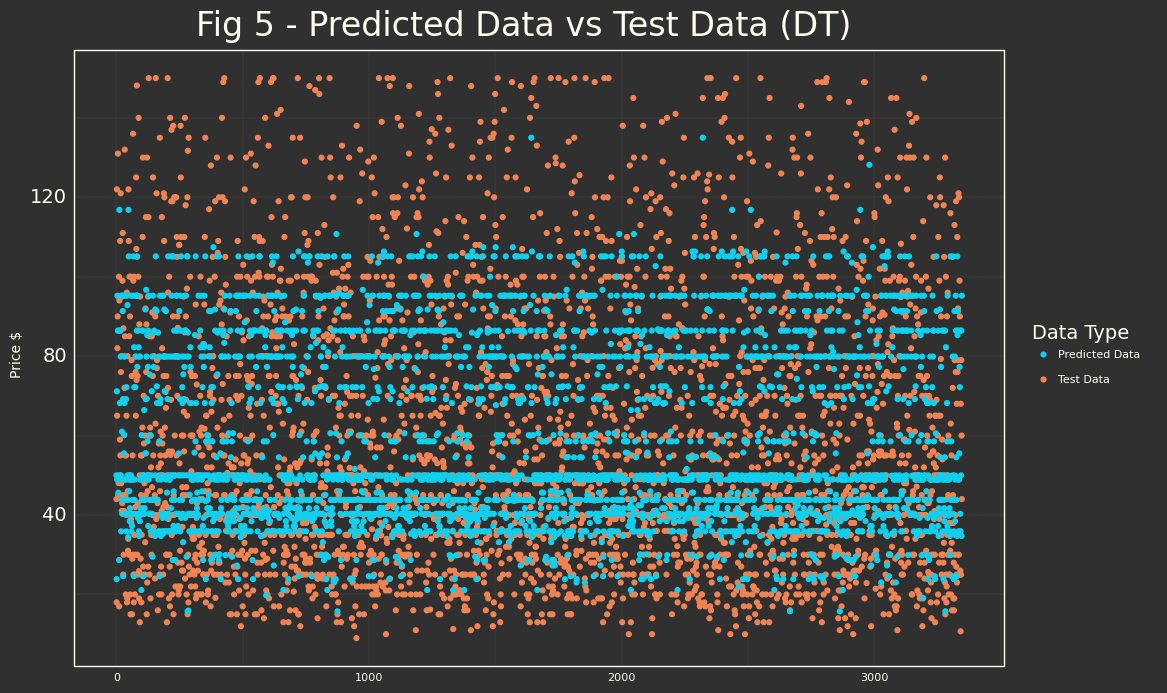It has aggregated some diferent prices classified by internal logic.

feat_imp=pd.DataFrame(dt.feature_importances_,
index=Xraw.columns,
columns=['Importance'])
feat_imp['feature'] = feat_imp.index
feat_imp_top10 = feat_imp.loc[feat_imp['Importance'].nlargest(10).index]
ax = sns.barplot(x="Importance", y="feature", data=feat_imp_top10,palette="Oranges")
# Title
plt.suptitle("Fig 6 - Decision Tree Top10 Features",y=0.93, size = 24);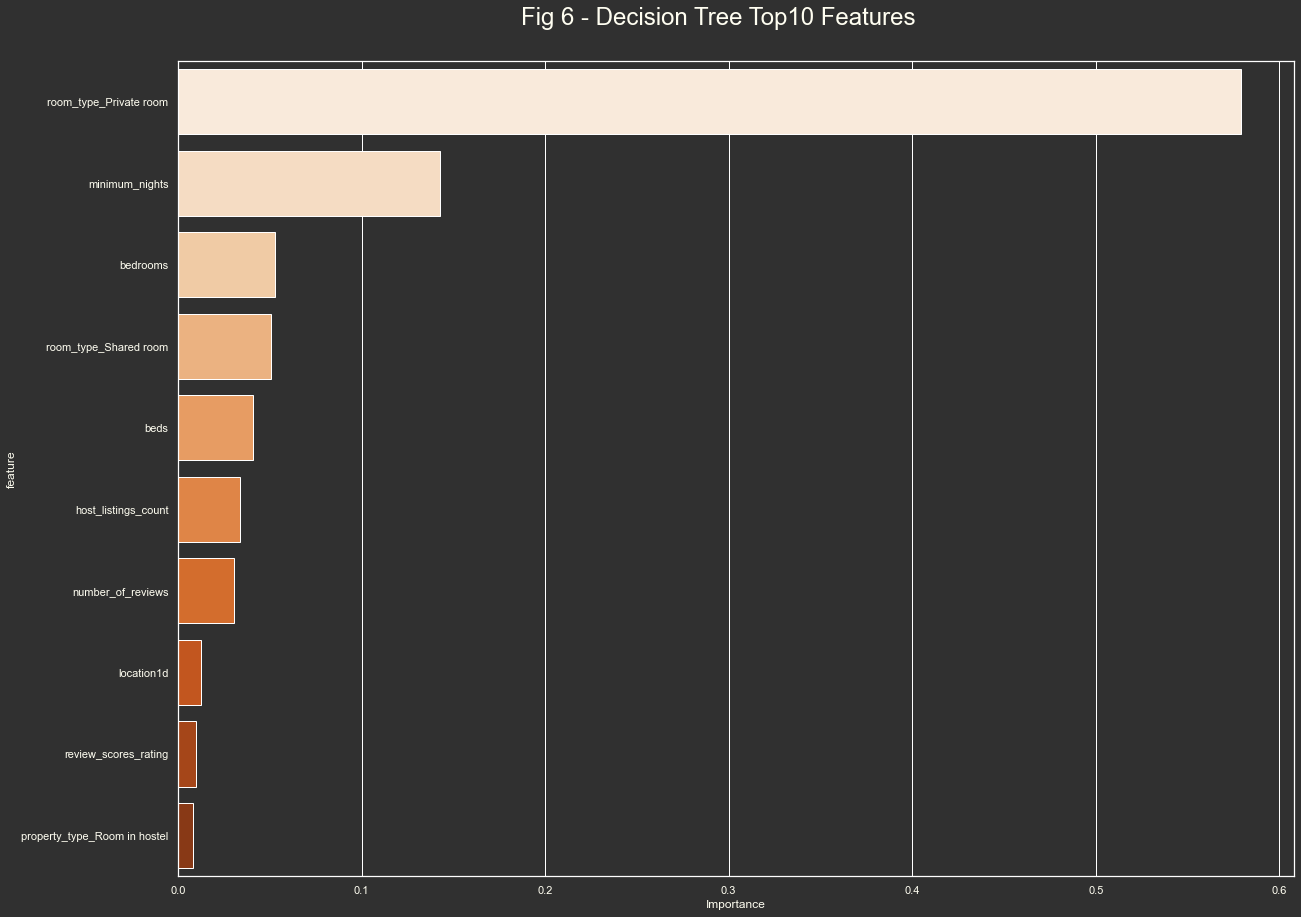## XGboost

xgb_round1 = XGBRegressor()

#ROUND1
param_grid = {
'learn_rate':[0.1,0.05,0.01],
'min_child_weight':[3,4,5],
'gamma':[i/10.0 for i in range(3,6)],
'subsample':[i/10.0 for i in range(6,11)],
'colsample_bytree':[i/10.0 for i in range(6,11)],
'max_depth': [9,10,11],
'n_estimators':[300,500,1000]
}

optimal_params = GridSearchCV(
estimator = xgb_round1,
param_grid=param_grid,
verbose=2, # NOTE: If you want to see what Grid Search is doing, set verbose=2
n_jobs = 2,
cv = 3
)

optimal_params.fit(X_train,
y_train,
early_stopping_rounds=10,
eval_set=[(X_test, y_test)],
verbose=True)

print(optimal_params.best_score_)
print(optimal_params.best_params_)

Fitting 3 folds for each of 6075 candidates, totalling 18225 fits

[Parallel(n_jobs=2)]: Using backend LokyBackend with 2 concurrent workers.
[Parallel(n_jobs=2)]: Done  37 tasks      | elapsed:   36.8s
[Parallel(n_jobs=2)]: Done 158 tasks      | elapsed:  2.5min
[Parallel(n_jobs=2)]: Done 361 tasks      | elapsed:  6.1min
[Parallel(n_jobs=2)]: Done 644 tasks      | elapsed: 10.8min
[Parallel(n_jobs=2)]: Done 1009 tasks      | elapsed: 16.9min
[Parallel(n_jobs=2)]: Done 1454 tasks      | elapsed: 24.6min
[Parallel(n_jobs=2)]: Done 1981 tasks      | elapsed: 33.5min
[Parallel(n_jobs=2)]: Done 2588 tasks      | elapsed: 43.3min
[Parallel(n_jobs=2)]: Done 3277 tasks      | elapsed: 56.9min
[Parallel(n_jobs=2)]: Done 4046 tasks      | elapsed: 70.5min
[Parallel(n_jobs=2)]: Done 4897 tasks      | elapsed: 84.9min
[Parallel(n_jobs=2)]: Done 5828 tasks      | elapsed: 100.7min
[Parallel(n_jobs=2)]: Done 6841 tasks      | elapsed: 117.8min
[Parallel(n_jobs=2)]: Done 7934 tasks      | elapsed: 137.0min
[Parallel(n_jobs=2)]: Done 9109 tasks      | elapsed: 159.0min
[Parallel(n_jobs=2)]: Done 10364 tasks      | elapsed: 183.0min
[Parallel(n_jobs=2)]: Done 11701 tasks      | elapsed: 212.9min
[Parallel(n_jobs=2)]: Done 13118 tasks      | elapsed: 242.8min
[Parallel(n_jobs=2)]: Done 14617 tasks      | elapsed: 274.6min
[Parallel(n_jobs=2)]: Done 16196 tasks      | elapsed: 318.1min
[Parallel(n_jobs=2)]: Done 17857 tasks      | elapsed: 355.4min
[Parallel(n_jobs=2)]: Done 18225 out of 18225 | elapsed: 363.5min finished

 validation_0-rmse:51.91973
Will train until validation_0-rmse hasn't improved in 10 rounds.
 validation_0-rmse:40.86495
Stopping. Best iteration:
    validation_0-rmse:23.95060

0.4834807959323069
{'colsample_bytree': 0.6, 'gamma': 0.3, 'learn_rate': 0.1, 'max_depth': 10, 'min_child_weight': 4, 'n_estimators': 300, 'subsample': 1.0}


#### Final XGBoost model after parameters

# Final model after adjusting parameters.
xg = XGBRegressor(seed=42,
objective='reg:squarederror',
gamma=0.3,
#learn_rate=0.05,
max_depth=10,
min_child_weight=4,
subsample=1,
colsample_bytree=0.6,
n_estimators=300)
xg.fit(X_train,
y_train,
verbose=False,
early_stopping_rounds=10,
eval_set=[(X_test, y_test)])

XGBRegressor(base_score=0.5, booster='gbtree', colsample_bylevel=1,
colsample_bynode=1, colsample_bytree=0.6, gamma=0.3, gpu_id=-1,
importance_type='gain', interaction_constraints='',
learning_rate=0.300000012, max_delta_step=0, max_depth=10,
min_child_weight=4, missing=nan, monotone_constraints='()',
n_estimators=300, n_jobs=0, num_parallel_tree=1, random_state=42,
reg_alpha=0, reg_lambda=1, scale_pos_weight=1, seed=42,
subsample=1, tree_method='exact', validate_parameters=1,
verbosity=None)

# XGB Regressor
train_predict=xg.predict(X_train)
test_predict=xg.predict(X_test)
xg_results=pd.DataFrame({'algorithm':['XGBoost'],
'Training error': [mean_absolute_error(y_train,train_predict)],
'Test error':[mean_absolute_error(y_test,test_predict)],
'Train_r2_score':[r2_score(y_train,train_predict)],
'Test_r2_Score':[r2_score(y_test,test_predict)]})
print(xg_results)

  algorithm  Training error  Test error  Train_r2_score  Test_r2_Score
0   XGBoost       12.439472   18.104947        0.749157       0.494361

result_df = pd.DataFrame({'Test Data':y_test,'Predicted Data':test_predict})
result_df.reset_index(drop=True,inplace=True)
result_df['id'] = result_df.index
result_df = pd.melt(result_df,id_vars='id', value_vars=['Test Data','Predicted Data'])
(
ggplot(result_df,aes(x='id',y='value',color='variable')) +
geom_point() +
scale_color_manual(values = ['#10D0EE',orange]) +
labs(title='Fig 7 - Predicted Data vs Test Data (XGB)',x='',y='Price $',color='Data Type') + )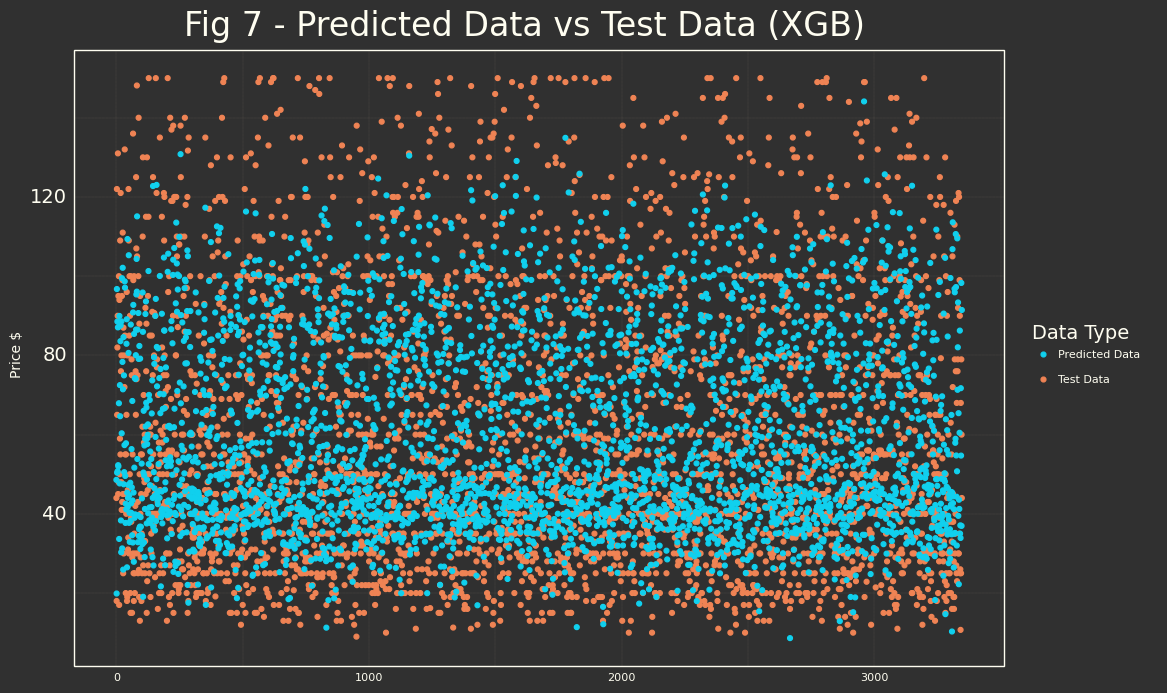feat_imp=pd.DataFrame(xg.feature_importances_, index=Xraw.columns, columns=['Importance']) feat_imp['feature'] = feat_imp.index feat_imp_top10 = feat_imp.loc[feat_imp['Importance'].nlargest(10).index] ax = sns.barplot(x="Importance", y="feature", data=feat_imp_top10,palette="Oranges") # Title plt.suptitle("Fig 8 - XGBoost Top10 Features",y=0.93, size = 24);import os import graphviz bst = xg.get_booster() node_params = {'shape': 'box', ## make the nodes fancy 'style': 'filled, rounded', 'fillcolor': '#FFE6C4:#FF9822'} leaf_params = {'shape': 'box', 'style': 'filled', 'fillcolor': '#ef8354:#FFB650'} graph_data = to_graphviz(booster=xg, num_trees=0, condition_node_params=node_params, leaf_node_params=leaf_params) source_graph = graph_data.source str(source_graph) texto = ''' graph [ rankdir=TB ] bgcolor="#303030" filesize="10,10" ''' source_graph = source_graph.replace('graph [ rankdir=TB ]',texto) source_graph = source_graph.replace('#0000FF','#10D0EE" fontcolor="#FFFEF1') source_graph = source_graph.replace('#FF0000','#FF0000" fontcolor="#FFFEF1') graph_data.source = source_graph graph_obj = graphviz.Source(graph_data.source) graph_obj.render(filename='airbnbBCN') graph_obj.view()##### Link to the Tree PDF ## Model Comparison pd.concat([rf_results,dt_results,xg_results],axis=0,ignore_index=True)  algorithm Training error Test error Train_r2_score Test_r2_Score 0 Random Forest 6.323665 17.327035 0.934937 0.529434 1 Decision Tree 18.362137 19.442400 0.477765 0.430368 2 XGBoost 12.439472 18.104947 0.749157 0.494361 The best model that it has been found using different technics is Random Forest. We will proceed to save it and we will be ready for consume it as a service. In terms of explaining the model, could be better to try to extract which is the information from xgboost, as it would easier to do some interpretation from this model than from Random Forest. ## Save the best model from joblib import dump, load dump(random_forest_regression, './models/randforest35_1000.joblilb')  ['./models/randforest35_1000.joblilb']  # Barcelona Airbnb DS analysis (4th part) \ |--Airbnb_Barcelona.ipynb | |--model |-randforestKK_NNNN.joblib  ## Deployment We could select some different ways to deploy (if required) our machine model. In part 3 we have saved joblib ML file and we can load our model from this file, and have 'just fitted' model to do our predictions. You can do different ways, create service with json interface to retrieve any calculation/classification etc... for this study I have tried to do it through streamlit, I have worked with plotly dash and some other ways of web deployment as flask or django and other dynamic presentation libraries as Bokeh and want to check how streamlit is to work with (I have liked ^^). So the code presented here is small streamlit dashboard. I have reduced the scope a lot to don't have 70 fields to fill etc... So I was only closing this project different way, learning a bit more. I have re-executed random forest regression with KBaseFilter of 7. Some of these fields could be grouped from a 'selection' point of view and the model has reduced its accuracy to 0.39, but I believe that for this case it's not important. Of course, if this web application is interesting for someone, we could improve a lot it, using full model, and adding some other features (neighbor comparison, error of the price predicted, etc...) ## DashBoard Code ### Import Libraries import streamlit as st import numpy as np import joblib from pathlib import Path path_directory = str(Path().absolute())  ### Define Title and constants Here we will start main() function. I use streamlit title widget and then I define my 'selection' values. I have taken them from unique values from original dataset. (df.column.unique). def main(): st.title('Airbnb Barcelona Advanced Analytics') beds_num = (0., 1., 2., 3., 4., 5., 6., 7., 8., 9., 10., 11., 12., 13., 14., 15., 16., 17., 18., 19., 20., 23., 24., 25., 28., 30., np.nan) bedrooms_num = ( 1., 2., 3., 4., 5., 6., 7., 8., 9., 10., 11., 12., 15., np.nan) Property_type = st.sidebar.selectbox("Room Type", ("Private Room", "Entire home/apt", "Entire Apartment", "Private Room in apartment", "Another")) host_listing_mean = 18.108853910477126  Then the logic of the widgets of selection and fill the array to use with the model.  if Property_type == 'Private Room': room_type_Private_room = 1 bedrooms_num = ( 1., np.nan) elif Property_type == 'Entire home/apt': room_type_Entire = 1 elif Property_type == 'Entire Apartment': property_type_Entire_apartment = 1 elif Property_type == 'Private Room in apartment': property_type_Private_room_in_apartment = 1 bedrooms_num = ( 1., np.nan) beds = st.sidebar.selectbox("Beds", beds_num) bedrooms = st.sidebar.selectbox("Bedrooms", bedrooms_num) host_listing_mean = 18.108853910477126 room_type_Entire = 0 room_type_Private_room = 0 property_type_Entire_apartment = 0 property_type_Private_room_in_apartment = 0 if Property_type == 'Private Room': room_type_Private_room = 1 elif Property_type == 'Entire home/apt': room_type_Entire = 1 elif Property_type == 'Entire Apartment': property_type_Entire_apartment = 1 elif Property_type == 'Private Room in apartment': property_type_Private_room_in_apartment = 1 # Price calculation ## Get the model file_path = path_directory + r"\models\randforest7_1000.joblilb" rf_model = joblib.load(file_path) X = [[bedrooms, beds, host_listing_mean, room_type_Entire, room_type_Private_room, property_type_Entire_apartment, property_type_Private_room_in_apartment]] y_predict = rf_model.predict(X)  Last aspects, some disclaimers and presentation.  st.sidebar.markdown(""" #### Notes For this explotation sample, random forest model has been reduced to 7 most important features. Some of them are different property types, Beds, Bedrooms and host_listing_counts, this one as it's something that is unknown for new properties has been reduced to the mean of the dataset. """) #Image st.image("wordcloud.png",use_column_width=True) # validation result = "{:.2f}".format(y_predict) st.success(f"Predicted Price= {result}$")


And usual execution function to package managing, etc...

if __name__ == "__main__":
main()


## Some Screenshots